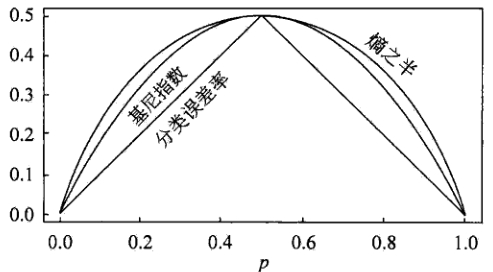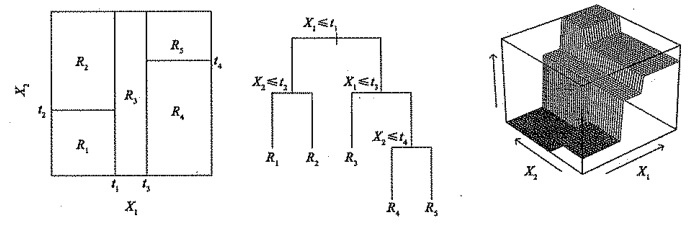# 深入理解机器学习——基于决策树的模型（一）：分类树和回归树

2 篇文章 4 订阅# 分类树

D = { ( x 1 , y 1 ) , ( x 2 , y 2 ) , . . . , ( x N , y N ) } D=\{(x_1, y_1), (x_2, y_2), ..., (x_N, y_N)\} 其中， x i = ( x i ( 1 ) , x i ( 2 ) , . . . , x i ( n ) ) T , x_i=(x_i^{(1)}, x_i^{(2)}, ..., x_i^{(n)})^T, 为输入实例，即特征向量， n n 为特征个数， i = 1 ， 2 … ， N i=1，2…，N N N 为样本容量， y i ∈ { 1 , 2 , . . . , K } y_i \in \{ 1, 2, ..., K\} 为类标。分类树学习的目标是根据给定的训练数据集构建一个决策树模型，使它能够对实例进行正确的分类。

\qquad 训练集： D = ( x 1 , y 1 ) , ( x 2 , y 2 ) , ⋯   , ( x N , y N ) D = {(x_1, y_1), (x_2, y_2), \cdots, (x_N, y_N)}
\qquad 属性集： A = a 1 , a 2 , ⋯   , a n A = {a_1, a_2, \cdots, a_n}

\qquad 函数 T r e e G e n e r a t e ( D , A ) TreeGenerate(D, A)

（ 1 ） 生成结点根node
（ 2 ） if D D 中样本全属于同一类别 C k C_k then
（ 3 ） \quad 将node标记为 C k C_k 类叶结点
（ 5 ） end if
（ 6 ） if A = ∅ A = \varnothing OR D D 中样本在 A A 上取值相同 then
（ 7 ） \quad 将node标记为叶结点，其类别标记为 D D 中样本数最多的类
（ 9 ）end if
（10）从 A A 中选择最优划分属性 a ∗ a_*
（11）for a ∗ a_* 的每一个值 a ∗ v a_*^v do
（12） \quad 为node生成一个分支：令 D v D_v 表示 D D 中在 a ∗ a_* 上取值为 a ∗ v a_*^v 的样本子集
（13） \quad if D v D_v 为空 then
（14） \qquad 将分支结点标记为叶结点，其类别标记为 D D 中样本最多的类
（17） \qquad T r e e G e n e r a t e ( D v , A − { a ∗ } ) TreeGenerate(D_v, A - \{a_*\}) 为分支结点
（19）end for

1. 当前结点包含的样本全属于同一类别，无需划分
2. 当前属性集为空，或是所有样本在所有属性上取值相同，无法划分
3. 当前结点包含的样本集合为空，不能划分

##### 决策树的优缺点
• 计算复杂度不高
• 对中间缺失值不敏感
• 解释性强，在解释性方面甚至比线性回归更强
• 与传统的回归和分类方法相比，决策树更接近人的决策模式
• 可以用图形表示，非专业人士也可以轻松理解
• 可以直接处理定性的预测变量而不需创建哑变量
• 决策树的预测准确性一般比回归和分类方法弱，但可以通过用集成学习方法组合大量决策树，显著提升树的预测效果

## 特征选择

### 信息增益

P ( X = x i ) = p i , i = 1 , 2 , ⋯   , n P(X = x_i) = p_i, i = 1, 2, \cdots, n

H ( X ) = − ∑ i = 1 n p i log ⁡ p i H(X) = -\sum_{i = 1}^n p_i \log p_i

H ( p ) = − ∑ i = 1 n p i log ⁡ p i H(p) = -\sum_{i = 1}^n p_i \log p_i

0 ≤ H ( p ) ≤ log ⁡ n 0 \leq H(p) \leq \log n

P ( X = 1 ) = p , P ( X = 0 ) = 1 − p , 0 ≤ p ≤ 1 P(X = 1) = p,\quad P(X = 0) = 1-p, \quad 0≤p≤1

H ( p ) = − p log ⁡ 2 p − ( 1 − p ) log ⁡ 2 ( 1 − p ) H(p) = -p \log_2 p - (1 - p)\log_2 (1 - p)p = 0 p = 0 p = 1 p = 1 H ( p ) = 0 H(p) = 0 ，随机变量完全没有不确定，当 p = 0.5 p = 0.5 时， H ( p ) = 1 H(p) = 1 ，熵取值最大，随机变量不确定性最大。

P ( X = x i , Y = y i ) = p i j { i = 1 , 2 , ⋯   , n j = 1 , 2 , ⋯   , m P(X = x_i, Y = y_i) = p_{ij} \quad \begin{cases} i = 1, 2, \cdots, n \\ j = 1, 2, \cdots, m \end{cases}

H ( Y ∣ X ) = ∑ i = 1 n p i H ( Y ∣ X = x i ) H(Y|X) = \sum_{i = 1}^n p_iH(Y|X = x_i)

g ( D , a ∗ ) = H ( D ) − H ( D ∣ a ∗ ) g(D, a_*) = H(D) - H(D|a_*)

1.计算数据集 D D 的经验熵 H ( D ) H(D) H ( D ) = − ∑ k = 1 K C k D log ⁡ 2 C k D H(D) = -\sum_{k=1}^K \frac{C_k}{D}\log_2\frac{C_k}{D}
2.计算特征 A A 对数据集 D D 的经验条件熵 H ( D ∣ A ) H(D|A) H ( D ∣ A ) = ∑ i = 1 n ∣ D i ∣ D H ( D i ) = − ∑ i = 1 n ∣ D i ∣ D ∑ k = 1 K D i k D i log ⁡ 2 D i k D i H(D|A) = \sum_{i=1}^n\frac{|D_i|}{D}H(D_i)=-\sum_{i=1}^n\frac{|D_i|}{D}\sum_{k=1}^K\frac{D_{ik}}{D_i}\log_2\frac{D_{ik}}{D_i}
3.计算信息增益： g ( D , a ∗ ) = H ( D ) − H ( D ∣ a ∗ ) g(D, a_*) = H(D) - H(D|a_*)

ID3算法的核心是在决策树各个结点上应用信息增益准则选择特征，递归地构建决策树。具体方法是：从根结点开始，对结点计算所有可能的特征的信息增益，选择信息增益最大的特征作为结点的特征，由该特征的不同取值建立子结点。之后，再对子结点递归地调用以上方法，构建决策树，直到所有特征的信息增益均很小或没有特征可以选择为止，最终得到一个决策树。ID3相当于用极大似然法进行概率模型的选择

ID3算法

1.若 D D 中所有实例属于同一类 C k C_k ，则 T T 为单结点树，并将类 C k C_k 作为该结点的类标记，返回决策树 T T
2.若 A = ∅ A = \varnothing ，则 T T 为单结点树，并将 D D 中实例数最大的类 C k C_k ，作为该结点的类标记，返回决策树 T T
3.否则，计算 A A 中各特征对 D D 的信息增益，选择信息增益最大的特征 a ∗ a_*
4.如果 a ∗ a_* 的信息增益小于阈值 ϵ \epsilon ，则置 T T 为单结点树，并将 D D 中实例数最大的类 C k C_k 作为该结点的类标记，返回决策树 T T
5.否则，对 a ∗ a_* 的每一可能值 a ∗ v a_*^v ，依 a ∗ = a ∗ v a_* = a_*^v D D 分割为若干非空子集 D v D_v ，将 D v D_v 中实例数最大的类作为标记，构建子结点，由结点及其子结点构成决策树 T T ，返回决策树 T T
6.对第 v v 个子结点，以 D v D_v 为训练集，以 A − { a ∗ } A - \{a_*\} 为特征集，递归地调用第(1)步~第(5)步，得到子树 T v T_v ，并返回子树 T v T_v

### 信息增益率

g g ( D , a ∗ ) = g ( D , a ∗ ) H ( D ) g_g(D, a_*) = \frac{g(D, a_*)}{H(D)}

C4.5算法

1.若 D D 中所有实例属于同一类 C k C_k ，则 T T 为单结点树，并将类 C k C_k 作为该结点的类标记，返回决策树 T T
2.若 A = ∅ A = \varnothing ，则 T T 为单结点树，并将 D D 中实例数最大的类 C k C_k ，作为该结点的类标记，返回决策树 T T
3.否则，计算 A A 中各特征对 D D 的信息增益和信息增益率，在信息增益大于 α \alpha 的特征中选择信息增益率最大的特征 a ∗ a_*
4.如果 a ∗ a_* 的信息增益率小于阈值 ϵ \epsilon ，则置 T T 为单结点树，并将 D D 中实例数最大的类 C k C_k 作为该结点的类标记，返回决策树 T T
5.否则，对 a ∗ a_* 的每一可能值 a ∗ v a_*^v ，依 a ∗ = a ∗ v a_* = a_*^v D D 分割为若干非空子集 D v D_v ，将 D v D_v 中实例数最大的类作为标记，构建子结点，由结点及其子结点构成决策树 T T ，返回决策树 T T
6.对第 v v 个子结点，以 D v D_v 为训练集，以 A − { a ∗ } A - \{a_*\} 为特征集，递归地调用第(1)步~第(5)步，得到子树 T v T_v ，并返回子树 T v T_v

#### 连续值处理

T a = { a i + a i + 1 2   ∣   1 ≤ i ≤ n − 1 } T_a = \{\frac{a^i + a^{i + 1}}{2} \ | \ 1 \leq i \leq n - 1\}

G a i n ( D , a ) = max ⁡ t ∈ T a G a i n ( D , a , t ) = max ⁡ t ∈ T a E n t ( D ) − ∑ λ ∈ { − , + } D t λ D E n t ( D t λ ) \begin{aligned} Gain(D, a) &= \max_{t \in T_a} Gain(D, a, t)\\ & = \max_{t \in T_a} Ent(D) - \sum_{\lambda \in \{-, +\}} \frac{D^\lambda _t}{D}Ent(D^\lambda _t) \end{aligned}

#### 缺失值处理

##### 划分属性的选择

ρ = ∑ x ∈ D ~ ω x ∑ x ∈ D ω x p ~ k = ∑ x ∈ D ~ k ω x ∑ x ∈ D ~ ω x r ~ v = ∑ x ∈ D ~ v ω x ∑ x ∈ D ~ ω x \begin{aligned} \rho & = \frac{\sum_{x \in \tilde{D}}\omega_x}{\sum_{x \in D}\omega_x}\\ \tilde{p}_k & = \frac{\sum_{x \in \tilde{D}_k}\omega_x}{\sum_{x \in \tilde{D}}\omega_x}\\ \tilde{r}_v & = \frac{\sum_{x \in \tilde{D}^v}\omega_x}{\sum_{x \in \tilde{D}}\omega_x} \end{aligned}

G a i n ( D , a ) = ρ × G a i n ( D ~ , a ) = ρ × ( E n t ( D ~ ) − ∑ x = 1 V r ~ v E n t ( D ~ v ) ) \begin{aligned} Gain(D, a) &= \rho \times Gain(\tilde{D}, a)\\ & = \rho \times (Ent(\tilde{D}) - \sum_{x = 1}^V \tilde{r}_v Ent(\tilde{D}^v)) \end{aligned}

### 基尼指数

G i n i ( D ) = ∑ k = 1 K p k ( 1 − p k ) = 1 − ∑ k = 1 K p k 2 Gini(D) = \sum^K_{k=1}p_k(1 - p_k) = 1 - \sum^K_{k=1}p_k^2

G i n i ( D ) = 2 p ( 1 − p ) Gini(D) = 2p(1 - p)

G i n i _ i n d e x ( D , a ) = ∑ v = 1 V D v D G i n i ( D v ) Gini\_index(D, a) = \sum^V_{v = 1}\frac{D^v}{D}Gini(D^v)

CART算法中，我们在候选属性集合 A A 中，选择那个使得划分后基尼指数最小的属性作为最优划分属性，即： a ∗ = arg min ⁡ a ∈ A G i n i _ i n d e x ( D , a ) a_* = \text{arg}\min_{a \in A}Gini\_index(D, a)## 分类树的剪枝

### 预剪枝

1. 定义一个高度，当决策树达到该高度时就停止决策树的生长
2. 达到某个节点的实例具有相同的特征向量，即使这些实例不属于同一类，也可以停止决策树的生长。这个方法对于处理数据的数据冲突问题比较有效。
3. 定义一个阈值，当达到某个节点的实例个数小于阈值时就可以停止决策树的生长
4. 定义一个阈值，通过计算每次扩张对系统性能的增益，并比较增益值与该阈值大小来决定是否停止决策树的生长。

### 后剪枝

#### 错误率降低剪枝（REP，Reduced-Error Pruning）

1. 删除以此结点为根的子树
2. 使其成为叶子结点
3. 当修剪后的树对于验证集合的性能不比修剪前的树的性能差时，则确认删除该结点，否则恢复该节点

#### 悲观错误剪枝（PEP，Pesimistic-Error Pruning）

∑ E i + 0.5 ∗ L ∑ N i \frac{\sum E_i +0.5 * L}{\sum N_i}

#### 代价复杂度剪枝（CCP，Cost-Complexity Pruning)

α = R ( t ) − R ( T t ) ∣ N t ∣ − 1 \alpha = \frac{R(t) - R(T_t)}{|N_t| - 1}

1. 对于完全决策树 T T 的每个非叶结点计算 α α 值，循环剪掉具有最小 α α 值的子树，直到剩下根节点，得到一系列的剪枝树 { T 0 , T ‘ 1 , T 2 , ⋯   , T m } \{ T_0, T_`1, T_2, \cdots, T_m \} ，其中 T 0 T_0 为原有的完全决策树， T m T_m 为根结点， T i + 1 T_{i +1} 为对 T i T_i 进行剪枝的结果
2. 从子树序列中，根据真实的误差估计选择最佳决策树
REPPEPCCP

# 回归树

1. 将预测变量空间（ X 1 , X 2 , X 3 , ⋯   , X p X_1, X_2, X_3, \cdots, X_p ）的可能取值构成的集合分割成 J J 个互不重叠的区域 { R 1 , R 2 , R 3 , ⋯   , R J } \{ R_1, R_2, R_3, \cdots, R_J\}
2. 对落入区域 R j R_j 的每个观测值作同样的预测，预测值等于 R j R_j 上训练集的各个样本取值的算术平均数。

R S S = ∑ j = 1 J ∑ i ∈ R j ( y i − y ^ R j ) 2 RSS = \sum^J_{j=1} \sum_{i \in R_j}(y_i - \hat{y}_{R_j})^2

R 1 ( j , s ) = { X ∣ X j < s } 和 R 2 ( j , s ) = { X ∣ X j ≥ s } R_1(j, s) = \{ X|X_j <s \} \quad \text{和} \quad R_2(j, s) = \{ X|X_j \geq s \}

∑ x i ∈ R 1 ( j , s ) ( y i − y ^ R 1 ) 2 + ∑ x i ∈ R 2 ( j , s ) ( y i − y ^ R 2 ) 2 \sum_{x_i \in R_1(j, s)}(y_i - \hat{y}_{R_1})^2 + \sum_{x_i \in R_2(j, s)}(y_i - \hat{y}_{R_2})^2## 回归树的剪枝

∑ m = 1 ∣ T ∣ ∑ x i ∈ R m ( y i − y ^ R m ) 2 + α ∣ T ∣ \sum_{m=1}^{|T|} \sum_{x_i \in R_m}(y_i - \hat{y}_{R_m})^2 + \alpha |T|

α \alpha 从0开始逐渐增加时，树枝以一种嵌套的、可预测的模式被修剪，因此获得与 α \alpha 对应的所有子树序列是很容易的。可以用交又验证或验证集确定 α \alpha ，然后在整个数据集中找到与之对应的子树：

1.利用递归二叉分裂在训练集中生成一额大树，只有当终端结点包含的观测值个数低于某个最小值时才停止。
2.对大树进行代价复杂性剪枝，得到一系列最优子树，子树是 α \alpha 的函数。
3.利用 K K 折交叉验诞选择 α \alpha 。具体做法是将训练集分为 K K 折。对所有 k = 1 , 2 , 3 , ⋯   , K k=1, 2, 3, \cdots, K ，对训练集上所有不属于第 k k 折的数据重复第(1)步~第(2)步得到与 α \alpha 对应的子树，并求出上述子树在第 k k 折上的均方预测误差。
4.每个 α \alpha 会有相应的 K K 个均方预测误差，对这 K K 个值求平均，选出使平均误差最小的 α \alpha
5.找出选定的 α \alpha 在第(2)步中对应的子树。

11-2945001-13640
02-15605
05-155万+
08-245507
09-262251
12-21611
07-214684
09-07564
05-277万+
05-271424
03-051160
05-11445
01-121万+
01-10992
08-203万+点击重新获取扫码支付余额充值